## Generating Synthetic Data Using Generative Adversarial Networks

Started 3 weeks ago by
Modified 3 weeks ago by
Views 189

Have you ever been in a situation where you need a dataset to try or showcase a new feature, present information externally or to other internal divisions, protect personally identifiable information before using data elsewhere, etc. I recently found myself in this situation, and anyone that's ever been in this situation knows that finding a good data set (especially if it needs to be legally approved) is a lot harder than it sounds. Given SAS's push towards generative A.I., I decided to use use a model that falls under the "generative A.I" umbrella, generative adversarial networks (also known as GANs) to tackle this task!  GANs can learn the joint probability distribution of a data set with different variable types to generate synthetic data that contains a distribution similar to the original dataset. In this post we’ll dive a deeper into the science behind GANs and will conclude the post with a demonstration on how to use the tabularGanTrain action to build GANs that allow you to generate synthetic data.

Generative Adversarial Networks (GANs) were first introduced by Ian Goodfellow and his colleagues in their paper "Generative Adversarial Nets" in 2014. The idea they introduced in this paper is what can we accomplish if we build two models that actively compete against one another? One of these models would be the generator, which captures the data distribution. The second model would be the discriminator (also known as critic) that estimates the probability of whether a sample came from the training data or the generator. The analogy the authors use to explain the model in layman's terms is that the generative model is analogous to a team of counterfeiters trying to produce fake currency and use it without detection. The discriminative model is analogous to the police trying to detect the counterfeit money.

As one can probably imagine, the objectives of these models are counter to one another. As a result, the training procedure for the generator is to maximize the probability of a discriminator making a mistake. On the other hand, the training procedure for the discriminator is to minimize the mistakes when comparing samples produced by the generator and the real distribution. Goodfellow et al proposed the use of fully connected networks for both the generator and the discriminator since this would allow the entire system to be parallelized and the networks can be trained with back propagation. Newer advancements in GANs modify the architecture of the system so that they use different types of networks and techniques. Two such advancements are pertinent to this post, the Conditional Tabular GAN (CTGAN) and the Correlation-Capturing GAN (CorGAN).

CTGAN

GANs can be used to generate a wide variety of data, such as image data, video data, audio data, and tabular data. Each type of data comes with its unique set of challenges, tabular data being no exception. To address the unique challenges posed by generating synthetic tabular data, the Conditional Tabular GAN (CTGAN) and the Correlation-Capturing GAN (CorGAN) were published in 2019 and 2020 and respectively. SAS combines concepts from both models to create the Correlation-Preserving Conditional Tabular GAN (CPCTGAN) model. This model is used in the tabularGanTrain action which I will showcase later in the post.

Some of the key challenges postured by Xu et al (CTGAN authors) in generating synthetic tabular data include the need to simultaneously model discrete and continuous columns, multi-model non-Gaussian values within continuous columns, learning from sparse one hot encoded vectors and imbalances in categorical columns. The CTGAN model introduces several novel techniques to address those issues, including augmenting the training produce with mode-specific normalization, architectural changes, a conditional generator to address data imbalances and training by sampling. In this post we will touch on these topics, but we will not address them exhaustively. For more information, please refer to the paper and GitHub .

Mode Specific Normalization

Discrete values can naturally be represented as one hot encoded vectors, but what about numeric columns with arbitrary distributions? Mode specific normalization helps CTGAN deal with columns that have complicated distribution. This method allows CTGAN to process the columns in the training dataset independently. The method can best be explained in three steps:

1. For each continuous column, a variational gaussian mixture model is used to estimate the number of modes and fit a gaussian mixture.
2. For each value in the continuous columns, compute the probability of each value coming from each node.
3. One mode is sampled from the given probability density and the sample mode is then used to normalize the value.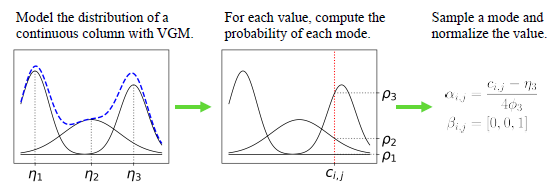Figure 1. Mode Specific Normalization .

Select any image to see a larger version.
Mobile users: To view the images, select the "Full" version at the bottom of the page.

The representation of a row then becomes the concatenation of continuous and discrete columns.

Conditional Generator and Training by Sampling

In a traditional GAN, vectors sampled from a standard multivariate normal distribution are fed into the model, however, this does not account for imbalances in the levels of categorical columns. Additionally, if the training data is randomly sampled, rows belonging to minor categories will be underrepresented. The goal is to resample in a way that all categories from discrete attributes are sampled evenly (but not necessarily uniform) during the training process and to recover the real data distribution during testing (not resampled). The solution consists of three key elements:

1. Conditional Vector: The conditional vector is introduced to indicate the condition that a discrete column takes on a specific value. The conditional vector is generated by concatenating various masks that represent one hot encoded vectors in discrete columns.
• e.g. We have two discrete columns, D1 = {1, 2, 3} and D2 = {1,2}. To represent the condition D2 = 1, we produce two mask vectors. These vectors are m1 = [0, 0, 0], which represents D1 in this case (no values from D1 selected), and m2 = [1, 0], which represents the fact that we want the first value in D2. Concatenating these two gives us the conditional vector [0, 0, 0, 1, 0].

1. Generator Loss: During training the conditional generator is free to produce any set of one-hot discrete vectors. The mechanism proposed to enforce the conditional generator to produce synthetic copies of the discrete columns is to add a penalty to the cross entropy loss function such that as the training advances, the generator learns to make copies of the one hot discrete vectors that match the training sets distributions.

1. Training by Sampling: The output produced by the conditional generator must be assessed by the discriminator. What the discriminator will do is that it is going to estimate the distance between the learned conditional distribution and the real data conditional distribution.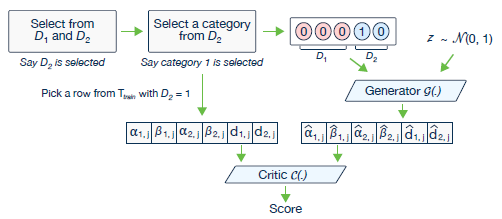Figure 2. CTGAN model/training by sampling method .

CTGAN Network Structure

The last major changes that allow the CTGAN models to work are the network structures of both the generator and the discriminator. Since columns in a row do not have any local structure, fully connected networks are used in both the generator and discriminator to attempt to capture all possible correlations between the columns. Furthermore, both models are trained using WGAN loss with a gradient penalty and ADAM optimization.Figure 3. Generator Network Architecture.Figure 4. Critic Network Architecture.

 Symbol Meaning ⨁ Concatenation ReLU Rectified Linear Unit activation function BN Batch Normalization FC Fully Connected layer tanh Hyperbolic Tangent activation function Gumbel Gumbel Softmax link function Leaky Leaky ReLU

Table 1. Legend for symbols used in architecture equations.

There’s a lot more that could be said regarding CTGAN and a lot of detail was left out of this post for the sake of brevity. For more information, please refer to the paper and GitHub in the references section .

CorGAN

CorGAN follows a lot of the ideas introduced by Goodfellow and the overall architecture is similar to CTGAN but there are some key differences:

1. A denoising autoencoder is trained and the generator output is passed through the decoder to generate the synthetic sample.
2. Unlike CTGAN, the input to the generator is only random noise and does not contain any real samples. This is true for both training and scoring.

The published autoencoder followed a very simple architecture where the encoder is simply a fully connected layer with a 128-dimensional output and tanh actication. The decoder also consists of a fully connected layer where the outputs are passed to a sigmoid function and the output dimensions match the training sample dimensions.

Just like CTGAN, a real sample and synthetic sample are then passed to the discriminator during training so that it’s able to learn whether an observation is real or synthetic. Due to the fact that we spent significant amount of time discussing CTGAN we will not be going in too much detail with regards to the CorGAN model/paper. For more information please refer to the paper and GitHub in the references section 

CPCTGAN

As stated earlier CPCTGAN, implements techniques from both the CTGAN and CorGAN models to generate synthetic tabular data. CPCTGAN combines the data transformation, conditional generator and training by sampling mechanisms from CTGAN. From CorGAN it adopts the mechanism to use a pre-trained autoencoder to then apply the decoder to the generator during model training.

SAS’ architecture for the autoencoder is also a lot more complex than the one published in the CorGAN GitHub repo for their proposed model.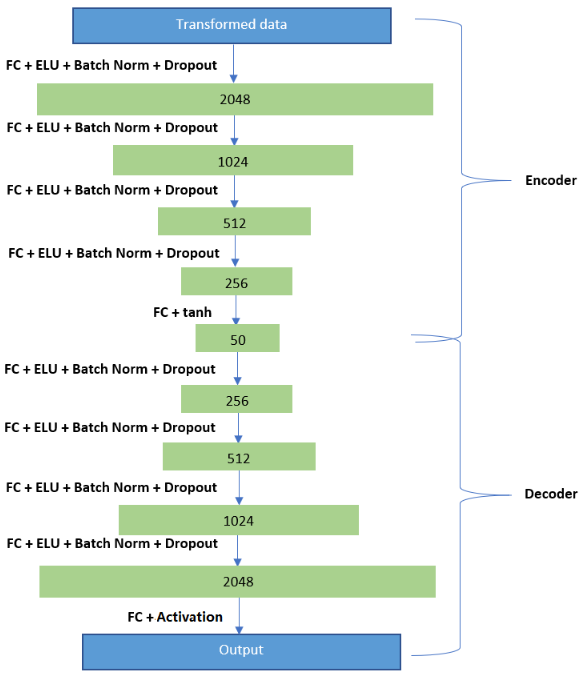Figure 5. CPCTGAN Autoencoder Architecture .

Since the autoencoder is also applied to the model architecture, overall it looks different than the previous two architectures.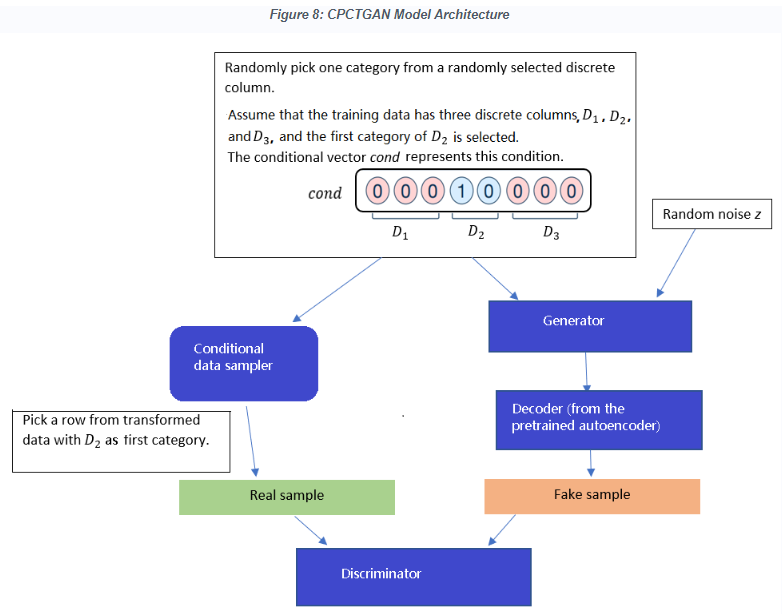Figure 6. CPCTGAN Entire Model Architecture .

Outside of the combination of those two models, there aren’t many additional differences between CPCTGAN and the other models.

tabularGanTrain Demonstration

This demonstration will be performed using Python; however, users should be aware that they can also use CASL and SAS Studio to perform everything in this demo. The first thing we need to do, like with most Python programs, is import the necessary packages:

``````# imports necessary packages
import os
import swat
import pandas as pd``````

 Package Use os Required to save files to disk swat Used to call the necessary CAS action sets, including the connection object and the model zoo actions. pandas Used to create dataframes/tables that can be saved in different formats.

Table 2. Python Package Description.

Now that we have imported the packages, we can then establish a connection to CAS using the SWAT’s CAS class and load the necessary action sets. In this demonstration I will load the dataPreprocess action set to impute missing values, the generativeAdversarialNet action set which allows us to train StyleGANs and CPCTGAN models, the percentile action set to assess models, and the sampling action set to partition data sets.

``````# Creates the connection object
conn = swat.CAS("connection information")

# Imports necessary action sets

To use the tabularGanTrain action all you need is the data set that you synthetically reacreate. In this demo I will use the home equity data set which is one commonly used around SAS. The link to download the dataset is listed in the reference. The data set contains information about customers such as their debt to income ratio, why they're requesting a home equity loan, their job category, if they've ever defaulted on a loan, etc. The data set has a target variable which states whether an individual defaulted on the loan or not.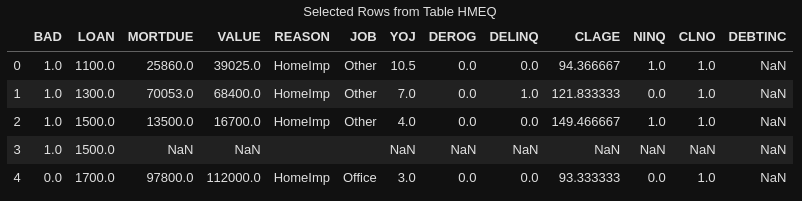Figure 7. HMEQ Sample.

As we can see in figure 7, there are a few data quality issues that need to be addressed. The dataset is mildly preprocessed, so there's not too much that we need to do. I performed simple imputation to replace missing values for the numeric and categorical columns. Additionally there are a few numeric variables with low cardinality that could be changed into categorical variables. Some categorical variables such as NINQ, DEROG, DELINQ, and CLNO were turned into binary categorical variables so that they simply indicate whether these events happen as opposed to the number of times that they occurred. The key reason for this is because these variables generally were very low cardinality with the majority of values taken being either 0 or 1. For that reason all categories that were greater than one were simply replaced with 1. The transformations aren't shown, but below you will see the necessary action in order for you to perform the imputation.

``````# Performs simple imputation on the continuous variables

conn.dataPreprocess.impute(
table              = "hmeq",
methodContinuous   = 'MEDIAN',
methodNominal      = 'MODE',
inputs             = inputs,
copyAllVars        = True,
casOut             = dict(name = "hmeq", replace = True)
)``````

Now that we have imputed our missing values we can proceed with generating the synthetic data by using the tabularGanTrain action. The options for the action include options for the optimization, number of samples that we want to generate, options for the gaussian mixture model, etc. In table 3 you will find the descriptions for the options that were used for this example.

``````# Creates the synthetic data

conn.tabularGanTrain(table = dict(name = "hmeq2", vars = inputs + ["BAD"]),
nominals      = nominals,
gpu           = {"useGPU":True,  "device":0},
miniBatchSize = 500,
seed          = 12345,
scoreSeed     = 1234,
numSamples    = 5960,
saveState     = {"name":"cpctStore", "replace":True},
casOut        = {"name":"synth_data",       "replace":True}
)``````

The task requires that we specify which variables are our inputs, and out of those inputs we need to specify which variables are categorical. The variables that we specify both in the vars option and nominals options are the ones that we are going to synthetically generate. In this demonstration, all of the variables (numeric and categorical) were synthetically generated. A total of 5,960 samples (number of rows in HMEQ) were synthetically generated. ADAM optimization was used for both the autoencoder and GAN, the Beta1, Beta2, alpha and learning rate were not changed. Each epoch of training uses 500 rows of data. There are a lot of settings that could be changed, but the results given these minimal changes were satisfactory.

 Option/Parameter Description table Name of the input table. Also used to specify the list of the inputs to be synthetically generated. nominals Specifies the name of the input variables that are nominal. gpu Specifies whether to use a GPU (if available) and which GPU (if multiple are available). optimizerAe Specifies the optimization settings for the autoencoder used in the model. optimizerGan Specifies the optimization settings for the GAN. miniBatchSize Number of observations used in each training iteration. seed Seed to use for the random number generator for training. scoreseed Seed used for the random number generator for scoring. numSamples Number of samples that will be synthetically generated. saveState Specifies the table in which to save the model state for model scoring. casOut Specifies the output CAS table in which to store the generated tabular data from the trained model

Table 3. tabularGanTrain options and parameters.

Model Assessment

Note that the action set has no way to accept a validation set. This makes sense considering there is no way to "how good" the synthetic data is. A simple way to start to assess the model is by  generating summary statistics for all of our columns.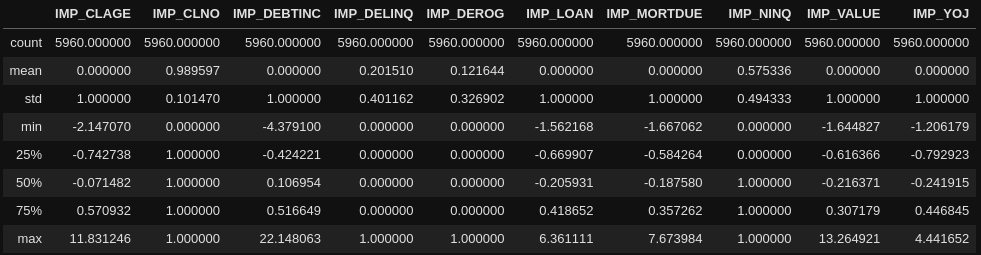Figure 8. Real Data Summary Statistics.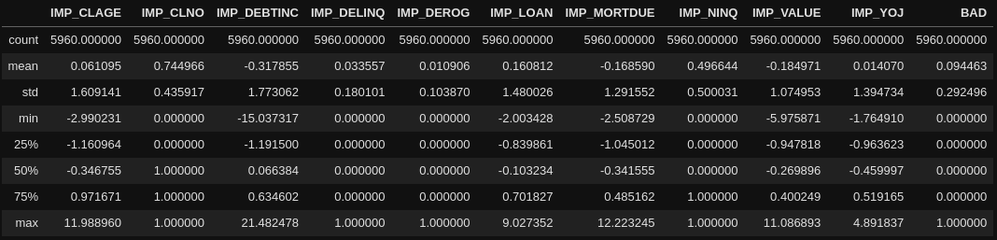Figure 9. Synthetic Data Summary Statistics.

As we can see the Summary statistics for both data sets look similar with some columns more than others (more on these observations later). There are multiple ways that we could potentially assess this data, one way we could assess the continuous variables could be by performing two sample t-tests to compare the means (comparing one real column mean to it's corresponding synthetic column mean). With regards to the categorical variables, we could perform tests of associations to measure whether there is a difference or not between the distributions of the levels. Although there are many ways to assess this model based on the use case, in this post the synthetic data set will be assessed in one of the ways proposed by Torfi and Fox in their CorGAN paper. This method is to train a predictive model using the real data, train a separate predictive model using the synthetic data, then assess both models against the real validation data set. Once the model predictions are generated, their performance can then be compared. Note that the model trained using synthetic data does not get any real data, even the target variable is synthetically generated. This assessment approach could be useful in a situation where synthetic data is being used in a machine learning context, although not every use case may benefit from assessing models using this approach.

In this post I will train gradient boosting models to assess the difference in performance between a model trained using the real data and one trained using the synthetic data. Both models were made using the same exact hyperparameter settings and the same training and validation partition sizes. Below you will find the code that was used to train both models:

``````# Creates a list of the dataset that will be used to create the models

datasets = ["hmeq", "synth_data"]

# Splits the original and synthetic datasets into training and validation

for data in datasets:
conn.sampling.srs(
table   = data,
samppct = 70,
seed = 919,
partind = True,
output  = dict(casOut = dict(name = data, replace = True),  copyVars = 'ALL')
)

# Trains a gradient boosting model using the original and synthetic datasets

for data in datasets:

model_name = "gboost_" + data

conn.decisionTree.gbtreeTrain(
table    = dict(name = data, where = '_PartInd_ = 1'),
target   = target,
inputs   = inputs,
nominals = nominals,
m        = 8,
nBins    = 100,
nTree    = 100,
casOut   = dict(name = model_name, replace = True)
)

# Scores both of the gradient boosting models

for data in datasets:

model_name = "gboost_" + data
output_tbl = "scored_" + data
assess_tbl = "assessed_" + data

conn.decisionTree.gbtreeScore(
table    = dict(name = "hmeq", where = '_PartInd_ = 0'),
model = model_name,
casout = dict(name=output_tbl,replace=True),
copyVars = target,
encodename = True,
assessonerow = True
)

conn.percentile.assess(
table = output_tbl,
casout = dict(name=assess_tbl,replace=True),
response = target,
event = "1"
)
``````

The final set of assessed tables contain information that can be used to construct ROC curves and lift curves. For this post, I decided to use accuracy and misclassification error to assess these models since the only goal here is binary classification. Choosing a probability cutoff of 0.5 the following accuracy and misclassification results were obtained: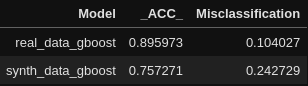Figure 10. Real Data vs. Synthetic Data Gradient Boosting Assessment (No Pre-processessing).

As we can see the gboost model trained with the real data has an accuracy that's approximately 14% better than the model trained using synthetic data. Overall for a model that was trained with purely synthetic data, the performance isn't bad. A small confession is that the results in figure 10 are for models that were trained before the continuous variables were standardized.

Observations and Tips

Observing the results generated by the GAN made me realize a few things that a user needs to be aware of:

1. The GAN does not recognize limits with numeric columns. E.G. In the real world, a borrower cannot receive a negative loan. Therefore, if a column has a lower limit of 0 (or an upper limit), the GAN will not be able to identify this and will generate values that exceed the limits.
2. The GAN will generate floating point values for continuous integer columns. Columns such as the number of times a borrower has gone delinquent on their loan will generally be represented with integer values and the number of unique values are often very low. As such it may be better to treat these columns as categorical. The key reason for these suggestions is because the GAN will generate values that may exceed the upper and or lower limits (as outlined in point 1) and two it will produce floating point values which may require the user to have to perform post-processing that will involve rounding/interpreting the numbers.
3. Performance seems to be worse on categorical columns, especially columns containing rare levels (a known issue addressed in  and ). For this reason it may be advantageous to bin categorical columns to reduce the overall amount of levels (especially rare levels). Other techniques such as weight of evidence encoding which can reduce the cardinality of your variables and convert them into numeric values may also improve the performance on categorical variables.
4. The GANs work seem to work much better on numeric columns with high means. It could be a function of the data considering that the variables in the data set with lower means also tended to have lower limits and lower cardinalities.

With all those observations in mind and out of the way, I wanted to perform an additional test and this is when I decided to normalize the numeric columns prior to generating the synthetic data. The main reason behind this approach was to make it so that our continuous columns fall along a normal distribution and can now generate better values (also negative outputs are not explicitly invalid). Better standardization techniques probably exist, but this was a quick one I could implement to test my observations. A downside of this approach is that it makes the model less interpretable, which could make this a harder decision to make in certain scenarios. After training the GAN and GBoost models using this new pre-processed data, there was an immediate increase in the performance of the model.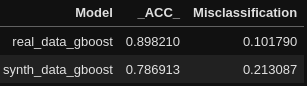Figure 11. Real Data vs. Synthetic Data Gradient Boosting Assessment (No Pre-processessing).

There are a few other ways that one could potentially improve this model. One of them is that there really isn't a limit with regards to how many synthetic observations the user could generate. For the sake of this demonstration both the real and synthetic training data set had the same amount of observations but it's possible that the sample used wasn't enough to completely represent the joint probability distribution of the data set. I took some steps to address some of the issues associated with the cardinality and distribution of certain variables, and I am sure that there are more ways to potentially preprocess these columns that could yield better performance. Different normalization techniques for the continuous columns is something that could also yield better results.

In conclusion, this technique can prove to be very useful whenever we would like to generate data with a similar distribution to protect personally identifiable information (PII), to present information to external organizations or other internal divisions, teaching courses, or anything in between. This technique could also be used as a data augmentation technique to enhance smaller training data sets and potentially improve the performance on validation. I'm sure there are many other ways someone could use this, and if you have more suggestions, I would love to know!

References:

Find more articles from SAS Global Enablement and Learning here.

Version history
Last update:
3 weeks ago
Updated by:Contributors
Article Labels